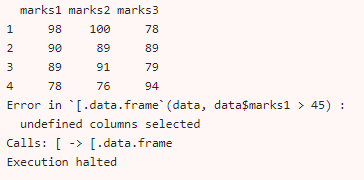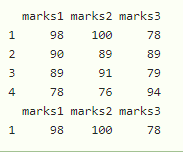# How to Handle “undefined columns selected” in R?

• Last Updated : 28 Nov, 2021

In this article, we will discuss how to handle “undefined columns selected” error in R Programming Language.

This error is specific to dataframe in R. This type of error will occur when we select a subset of a data frame and forget to add a comma.

Example: Check the error in the dataframe

Here we created a dataframe with 3 columns and select the values in which the second column value is greater than 45

## R

 `# create dataframe with 4 rows and 3 columns``data = ``data.frame``(marks1=``c``(98, 90, 89, 78), ``                  ``marks2=``c``(100, 89, 91, 76), ``                  ``marks3=``c``(78, 89, 79, 94))`` ` `# display``print``(data)`` ` `# now select values from marks2 column ``# which are greater than 45``data[data\$marks1 > 45]`

Output:This is because of neglecting comma(,) behind the value. The dataframe must choose the column after comma operator. So we have to keep comma.

Example:

## R

 `# create dataframe with 4 rows and ``# 3 columns``data = ``data.frame``(marks1=``c``(98, 90, 89, 78),``                  ``marks2=``c``(100, 89, 91, 76),``                  ``marks3=``c``(78, 89, 79, 94))`` ` `# display``print``(data)`` ` `# now select values from marks2 column ``# which are greater than 90``data[data\$marks1 > 90, ]`

Output:My Personal Notes arrow_drop_up